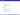# Create presentations in Perl 6 Pod

Perl 6 Pod is awesome markup language. I use the Pod as often as possible. For example, for creating presentations. How am I doing this?

I open my favirite text editor and start presentation with а DESCRIPTION:

 =for DESCRIPTION :title('presentation template in Perl 6 Pod format') = :author('Aliaksanr Zahatski') :pubdate('2011') 

The named blocks =Slide are used to describe each presentation screen:

 B<=begin Slide :title('About me')> http://search.cpan.org/$\sim$zag/ lang/rakudo ( FreeBSD ) Russian book "Everything about Perl 6" B<=end Slide> 

Lists are specified as a series of =item blocks :

 =begin Slide :title('Content') key moments: =item features =item implementations =item usage =end Slide 

A =Image block is used for insert pictures:

 =for Image :width('3in') i/si26.jpg 

The =config directive allows you to prespecify default images size:

 =config Image :width('2in') 

For specifying the code samples use standart Pod block with attribute lang:

 =for code B<:lang('Perl')> my @keys = <a b c d e>; my @vals = ^5; my %hash = @keys Z @vals; 

or

 =begin code B<:lang('JavaScript')> var keys = ['a', 'b', 'c']; var values = [1, 2, 3]; var map = {}; //this is a comment for(var i in keys) { map[ keys[i] ] = values[i]; } =end code 

would be rendered:The following example demonstrates the use of pauses:

 =begin Slide :title('Perl6::Pod::Slide: pause demo') Now : B<=Pause> =for item :numbered Lists =for item :numbered B<:pause> Tables =for item :numbered Headers =end Slide 

Use =Latex to type Latex text;

 =Latex $\Gamma_0 S_0 (1-\alpha)^2 = k_d \alpha^2 \quad \Rightarrow \quad \Bigl(\frac{1-\alpha}{\alpha}\Bigr)^2 = \frac{k_d}{\Gamma_0 S_0}$To insert a vector graphics I use coneverter epstopdf, and then insert it with =Image block.

Fork presentation template  designed for easy start and type "make" to build pdf file.

You will need the following software to be installed: latex-beamer, Perl 5 and Perl6-Pod-Slide .

 Presentation template in Perl 6 Pod format.https://github.com/zag/template-pod6

 Perl6::Pod::Slide - make slides easy. http://search.cpan.org/perldoc?Perl6::Pod::Slide